# Constructive t-secure Homomorphic Secret Sharing for Low Degree Polynomials

Introduction
• Homomorphic secret sharing is a way to outsource the computation to a set of servers while restricting some subsets of servers from learning about the secret inputs.
• We will consider the setting as shown in the diagram. There are input clients, and each has its secret input. The input clients secretly share the inputs to the computing servers. The goal of the scheme is to let the output client learn a function of the inputs. For security, no one should know the input except its owner.
• Homomorphic secret sharing can be seen as a multi-party computation protocol with small number of communications. For the applications, it enables secure computation on cloud servers where inputs must be kept secret. It also enables secure machine learning where the training data and models may be sensitive.
•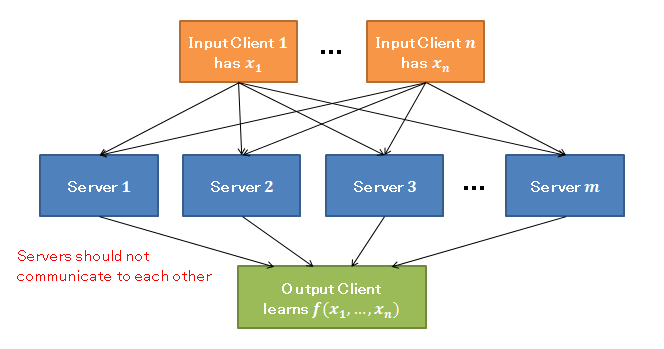Contributions
• In the previous work, Lai et al. [LMS18] proposed a homomorphic secret sharing scheme based on homomorphic encryption. However, it is only 1-secure. This means any subsets of servers should not collude at all, including any pairs of servers, which is not a realistic situation. And moreover, in their suggested t-secure scheme, where at most t servers can collude, the scheme requires solving NP-hard problems, and requires t square servers.
• In this work, we propose t-secure homomorphic secret sharing scheme for low degree polynomials that does not require solving NP-hard problem, and there is no number of required servers. We use the idea of secret sharing from Ito et al. [ISN87].
•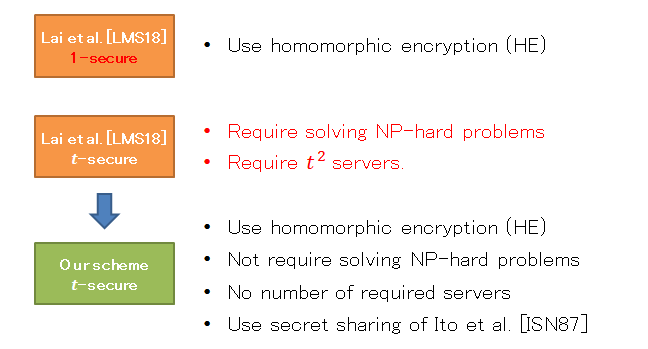[LMS18] Lai R.W.F, Malavolta G, Schroder D, "Homomorphic secret sharing for low degree polynomials," Asiacrypt, 2018.
[ISN87] Ito M, Saito A, Nishizeki T, "Secret sharing schemes realizing general access structure," IEEE Globecom, 1987.
Simplified example
• Suppose that there are two input clients with secret inputs a and b. The goal is to let the output client learns the value a times b. We build the scheme on degree 1 homomorphic encryption (allow linear operations on ciphertexts) and use 3 computing servers.
• If we do not want any pair of servers to learn the input, the maximal non-access structure will be as shown in the figure.
• Non-access set is a subset of servers that is not allowed to reconstruct the secret. Non-access structure is a set that contains all chosen non-access sets. The maximal non-access structure contains all non-access sets that are not subset of other non-access sets.
•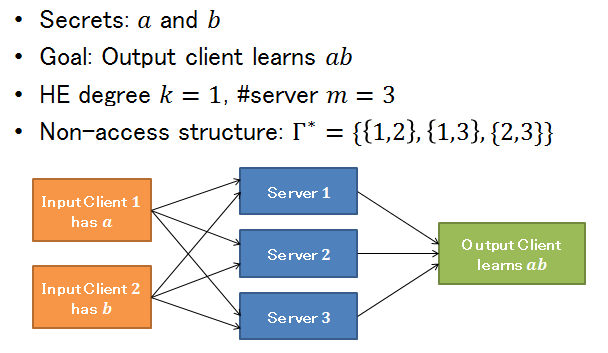• In the first step, input clients generate their shares. Inputs a and b are divided into 3 parts. The sum of shares are equal to a and b. Next, the shares are distributed where the j-th server gets the share a_gamma and b_gamma as ciphertexts if j is in gamma, otherwise it gets as plaintexts. Now, each computing server gets 6 shares, four of them are ciphertexts, and two of them are plaintexts. Note that the ciphertexts are marked with boxes. It can be seen that without secret key, combining shares from only two servers will not violate the security. While combining shares from all servers will reveal the secret inputs a and b.
•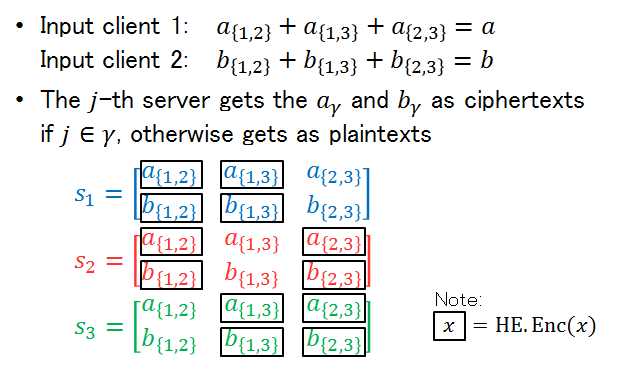• After secret sharing, each server performs local computation on the shares. The goal is to compute a times b which is a polynomial degree 2 of inputs. The function a times b is divided into 3 parts, each part for each server. Server 1 will locally compute y_1 as shown in the figure. Server 2 and 3 also locally compute y_2 and y_3. Then, y_1, y_2, and y_3 are sent to the output client. After adding all shares together and then decrypt, the output client learns the value a times b. It can be seen that the calculation for each server and the output client only requires homomorphic encryption of degree 1.
•Results
• We consider these four parameters.
•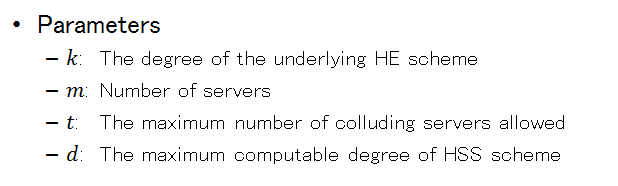• Firstly, we increase the maximum degree of Shamir's homomorphic secret sharing scheme [Sha79]. Comparing to the classic Shamir's scheme which is information theoretically secure and does not base on homomorphic encryption, our scheme with homomorphic encryption has around k times increasing in the degree of the homomorphic secret sharing. The security models are both threshold-t.
• Secondly, we add threshold structures to the work of Lai et al. [LMS18]. Our work supports any value of threshold. The final degree that our scheme can compute based on degree-k homomorphic encryption is shown in the bottom right. Comparing to the work of Lai et al. [LMS18], our final degree has a division of t as a trade-off with security.
• Thirdly, we decrease the number of required servers in t-secure scheme of Lai et al. [LMS18]. In their work only one setting of t-secure scheme is suggested. They consider the number of servers as a result, while the other parameters are requirements. Comparing to t square servers in the their work, our scheme do not have a restriction on the number of servers.
• Finally, we extend the capability of homomorphic encryption. Comparing to the setting using only homomorphic encryption, we mitigate the single point of failure by adding more servers to the scheme, and also increase the computable degree at the same time.
• It can be seen that the number of shares of our scheme seems to be large. Please see more discussion in the paper.
•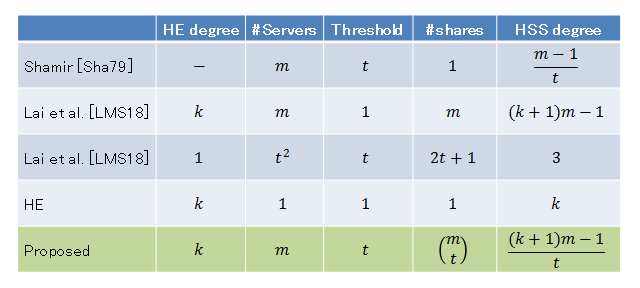[Sha79] Shamir A, "How to share a secret," Commun. ACM, 1979.
[LMS18] Lai R.W.F, Malavolta G, Schroder D, "Homomorphic secret sharing for low degree polynomials," Asiacrypt, 2018.